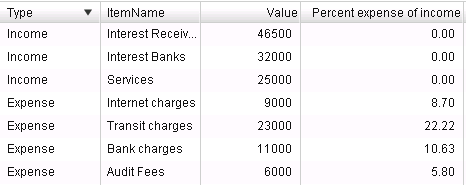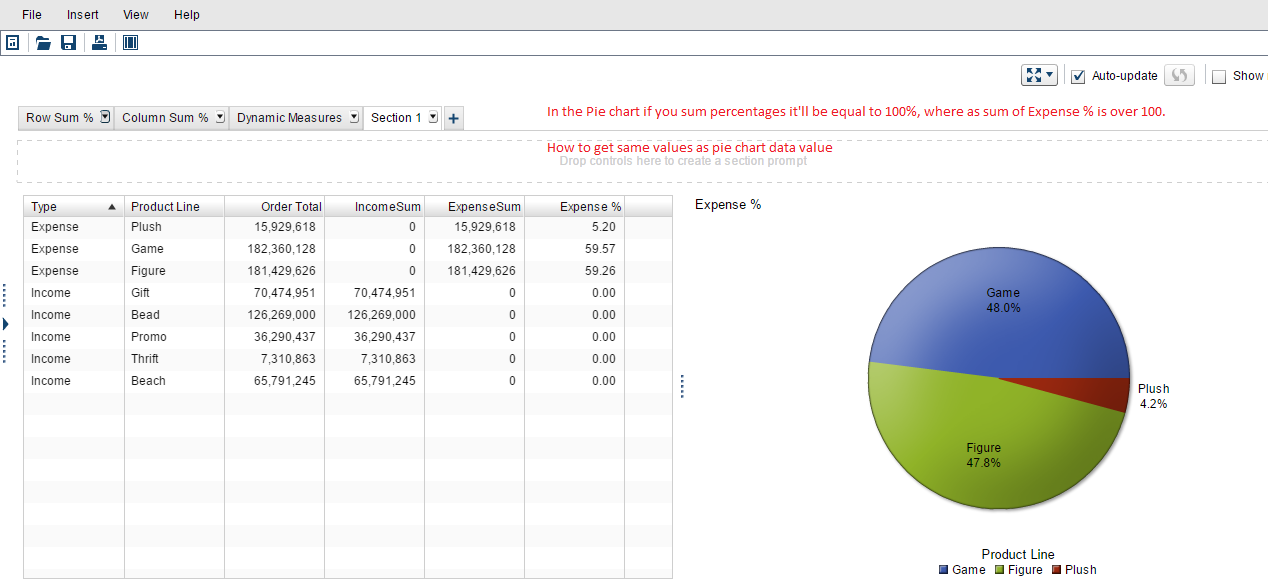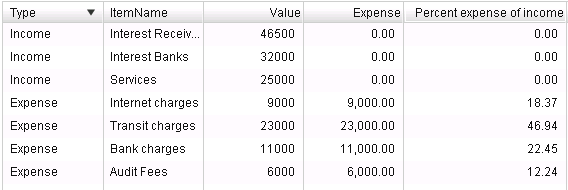## Percentage of Expenses from Total Income: SAS VA

Hi Community,

I'm using SAS VA 7.2 my sample data is as follows:

Type          ItemName              Value

Income      Services                  25000

Income      interest -banks        32000

Expense    Transit charges       23000

Expense    Internet charges      9000

Expense    Audit Fees               6000

Expense    Bank charges          11000

How can I calculate Percentage of each Expense from Total Income?

like:

% Transit charges from Total Income=?

% Internet charges from Total Income=? and so on...

Regards,

Balraj

5 REPLIES 5

## Re: Percentage of Expenses from Total Income: SAS VA

Hi## Re: Percentage of Expenses from Total Income: SAS VA

Yes Spot on..!!! @ShivamKS,

I've implemented till what you've implemented, was about to update that.

Now I'm struck at this point, let me explain:

let suppose sum percent expenses of income =47.35

where as what i'm trying to achieve is it should be equal to 100% of expenses

i.e., sum percent expenses of income = 100% = Total Expenses %

This is what i'm able to achieve:## Re: Percentage of Expenses from Total Income: SAS VA

In the pie chart that values are % of total expense and not % of total income, that is why when you total them they give 100%.

They are two different calculations isn't it? Not sure if i am missing something.

## Re: Percentage of Expenses from Total Income: SAS VA

Hi

In order to get values same as pie chart like:The calculation:## Re: Percentage of Expenses from Total Income: SAS VA

Wow Thank you very much @ShivamKS now I've understood how it is working internally.

And I've also understood that SUM of % of Expense from Total Income will not be equal to 100%, as it is calculating separately for

each group.

Is there any alternate method to achieve the same?

Please share some inputs on this.

Discussion stats
• 5 replies
• 1406 views
• 2 likes
• 2 in conversation